#A REVIEW OF LOGARITHMS## Equivalence

Before you choose a topic from the list, let's review what you know about the word "equivalent." You will come across this word in all your math courses. According to Webster, the word "equivalent" means to have equal value, equal amount, equal measure, equal area, equal length, etc. You get the picture.
For example, 1 yard is equivalent to 3 feet because both represent the same measure. You will see the notation 1 yard = 3 feet. Obviously, the left side of the = sign is not identical to the right side. The numbers and the words are different. However, the left side is equivalent to the right side because both sides denote the same length. In this case, the = sign is used to denote equivalence.
Suppose you wish to purchase an item for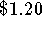, you could pay for it with a dollar bill and 2 dimes, you could pay for it with 12 dimes, you could pay it for 4 quarters and 4 nickels, etc. If you put each method of payment in a separate pile, you will note that they do not look the same. They are in fact not identical. However, they are equivalent because all the methods of payment total.

The number 1 has many equivalent faces: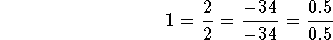to name a few. The number 0 has many equivalent faces: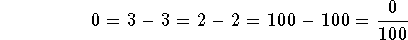to name a few.

## Exponential Shortcuts

Exponents represent shortcuts in multiplication.

Example 1: The expression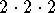can be written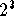.

Example 2: The expression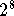means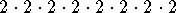.

Example 3: The expression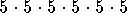can be written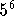.

Example 4: The expression (-7)(-7)(-7)(-7) can be written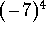.

You would much rather write the expression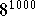than write out 8 one thousand times. You can also use exponents with variables.

Example 5: The expression (a+b)(a+b)(a+b)(a+b)(a+b) is more conveniently written as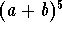.

If you want to review the rules for exponents, click on Exponential Rules.[Exponential Rules] [Logarithms]

[Algebra] [Trigonometry ] [Complex Variables]S.O.S MATHematics home page

Do you need more help? Please post your question on our S.O.S. Mathematics CyberBoard.Author: Nancy Marcus